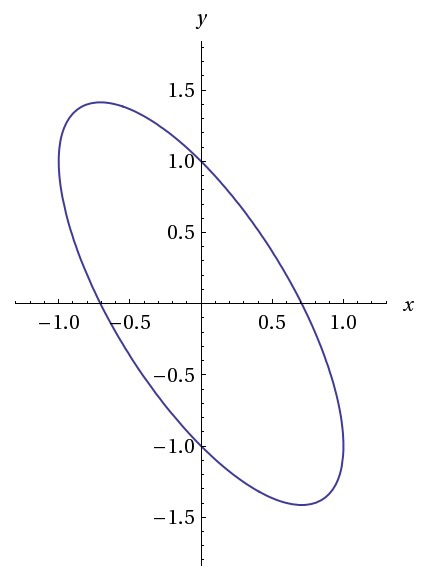# It's a jelly bean!

Geometry Level 2The graph above shows the figure created by the function $x^2+(y+x)^2=1$.

Find the area of this tilted ellipse correct to 2 decimal places.

×